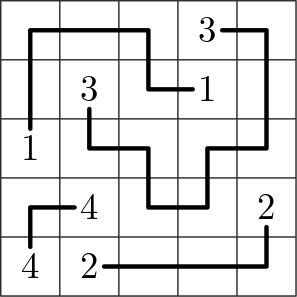A single player game in which the player connects pairs of points in a rectangular grid with paths traversing all the grid cells.## Description

Zig-Zag Numberlink is a single player game played on a rectangular grid. Initially, some of the cells of the grid are empty while others contain a number from $1$ to $k$, each of which appears exactly twice.

Each grid cells is adjacent to the cells to its left, right, top, and bottom, if any. The goal is to find a collection of $k$ simple paths (sequences of adjacent cells) such that: (i) the endpoints of the $i$-th path are the two cells containing number $i$, and (ii) each cell of the grid belongs to exactly one path.

A popular smartphone game based on Zig-Zag Numberlink is Flow Free.

## Computational complexity

The problem of deciding whether an instance of Zig-Zag Numberlink admits a solution is NP-Complete .

The variant in which each path is required to use the minimum number of turns when considering the other paths as fixed is known as Numberlink.

 A. Adcock, E. D. Demaine, M. L. Demaine, M. P. O’Brien, F. Reidl, F. S. Villaamil, B. D. Sullivan, “Zig-Zag Numberlink is NP-Complete”, Journal of Information Processing, 2015.

@misc{cog:zig-zag-numberlink,
author = "{CoG contributors}",
title  = "{Zig-Zag Numberlink (Flow Free) --- Complexity of Games}",
year   = "2022",
}